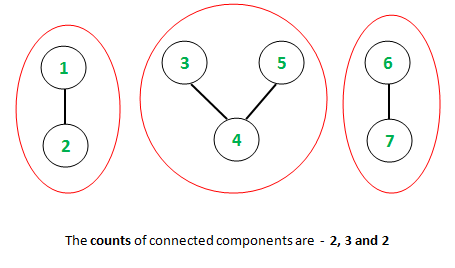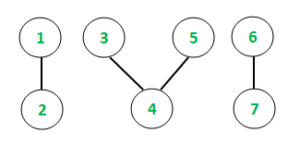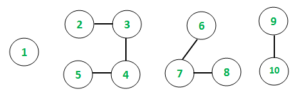# Count of unique lengths of connected components for an undirected graph using STL

Given an undirected graph, the task is to find the size of each connected component and print the number of unique sizes of connected componentsAs depicted above, the count(size of connected component) associated with the connected components are 2, 3 and 2. Now, the unique count of the components are 2 and 3. Hence the expected result is Count = 2

Examples:

```Input: N = 7Output: 1 2 Count = 2
3 4 5 Count = 3
6 7 Count = 2
Unique Counts of connected components: 2

Input: N = 10Output: 1 Count = 1
2 3 4 5 Count = 4
6 7 8 Count = 3
9 10 Count = 2
Unique Counts of connected components: 4
```

## Recommended: Please try your approach on {IDE} first, before moving on to the solution.

Prerequisites: Depth First Search

Approach:
The basic idea is to utilize the Depth First Search traversal method to keep a track of the connected components in the undirected graph. An STL container Set is used to store the unique counts of all such components since it is known that a set has the property of storing unique elements in a sorted manner. Finally, extracting the size of the Set gives us the necessary result. The step-wise implementation is as follows:

1. Initialize a hash container (Set), to store the unique counts of connected components.
2. Recursively call Depth First Search traversal.
3. For every vertex visited, store the count in the set container.
4. The final size of the Set is the required result.

Below is the implementation of the above approach:

## C++

 `// C++ program to find unique count of ` `// connected components ` `#include ` `using` `namespace` `std; ` ` `  `// Function to add edge in the garph ` `void` `add_edge(``int` `u, ``int` `v, vector<``int``> graph[]) ` `{ ` `    ``graph[u].push_back(v); ` `    ``graph[v].push_back(u); ` `} ` ` `  `// Function to traverse the undirected graph ` `// using DFS algorithm and keep a track of ` `// individual lengths of connected chains ` `void` `depthFirst(``int` `v, vector<``int``> graph[], ` `                ``vector<``bool``>& visited, ``int``& ans) ` `{ ` `    ``// Marking the visited vertex as true ` `    ``visited[v] = ``true``; ` `    ``cout << v << ``" "``; ` ` `  `    ``// Incrementing the count of ` `    ``// connected chain length ` `    ``ans++; ` ` `  `    ``for` `(``auto` `i : graph[v]) { ` `        ``if` `(visited[i] == ``false``) { ` `            ``// Recursive call to the DFS algorithm ` `            ``depthFirst(i, graph, visited, ans); ` `        ``} ` `    ``} ` `} ` ` `  `// Function to initialize the graph ` `// and display the result ` `void` `UniqueConnectedComponent(``int` `n, ` `                              ``vector<``int``> graph[]) ` `{ ` ` `  `    ``// Initializing boolean visited array ` `    ``// to mark visited vertices ` `    ``vector<``bool``> visited(n + 1, ``false``); ` ` `  `    ``// Initializing a Set container ` `    ``unordered_set<``int``> result; ` ` `  `    ``// Following loop invokes DFS algorithm ` `    ``for` `(``int` `i = 1; i <= n; i++) { ` `        ``if` `(visited[i] == ``false``) { ` `            ``// ans variable stores the ` `            ``// individual counts ` `            ``int` `ans = 0; ` ` `  `            ``// DFS algorithm ` `            ``depthFirst(i, graph, visited, ans); ` ` `  `            ``// Inserting the counts of connected ` `            ``// components in set ` `            ``result.insert(ans); ` `            ``cout << ``"Count = "` `<< ans << ``"\n"``; ` `        ``} ` `    ``} ` ` `  `    ``cout << ``"Unique Counts of "` `         ``<< ``"connected components: "``; ` ` `  `    ``// The size of the Set container ` `    ``// gives the desired result ` `    ``cout << result.size() << ``"\n"``; ` `} ` ` `  `// Driver code ` `int` `main() ` `{ ` `    ``// Number of nodes ` `    ``int` `n = 7; ` ` `  `    ``// Create graph ` `    ``vector<``int``> graph[n + 1]; ` ` `  `    ``// Constructing the undirected graph ` `    ``add_edge(1, 2, graph); ` `    ``add_edge(3, 4, graph); ` `    ``add_edge(3, 5, graph); ` `    ``add_edge(6, 7, graph); ` ` `  `    ``// Function call ` `    ``UniqueConnectedComponent(n, graph); ` ` `  `    ``return` `0; ` `} `

Output:

```1 2 Count = 2
3 4 5 Count = 3
6 7 Count = 2
Unique Counts of connected components: 2
```

Time Complexity:
As evident from the above implementation, the graph is traversed using the Depth First Search algorithm. The individual counts are stored using Set container wherein the insertion operation takes O(1) time. The overall complexity is solely based on the time taken by the DFS algorithm to run recursively. Hence, the time complexity of the program is O(E + V) where E is the number of edges and V is the number of vertices of the graph.
Auxiliary Space: O(N)My Personal Notes arrow_drop_upCheck out this Author's contributed articles.

If you like GeeksforGeeks and would like to contribute, you can also write an article using contribute.geeksforgeeks.org or mail your article to contribute@geeksforgeeks.org. See your article appearing on the GeeksforGeeks main page and help other Geeks.

Please Improve this article if you find anything incorrect by clicking on the "Improve Article" button below.

Be the First to upvote.

Please write to us at contribute@geeksforgeeks.org to report any issue with the above content.